﻿ 平台经济下的安全监管：以顺风车市场整改为例
>《财经研究》
2022第48卷第5期

1. 上海财经大学 商学院, 上海 200433;
2. 俄克拉荷马大学 经济系, 美国 诺曼 73019;
3. 上海理工大学 管理学院, 上海 200093

Safety Supervision under the Platform Economy: Taking the Ride-hailing Market as an Example
Zou Gan1, Liu Qihong2, Wang Xiaofang3
1. School of Business, Shanghai University of Finance and Economics, Shanghai 200433, China;
2. Department of Economics, University of Oklahoma, Norman 73019, the United States;
3. School of Business, University of Shanghai for Science and Technology, Shanghai 200093, China
Summary: Taking the rectification policy of the ride-hailing platform as an example, this paper analyzes the effectiveness of two control methods — “driver access threshold” and “platform safety construction”. First, based on the maximization of platform profits and total social welfare, the differences in action incentives under platform autonomy and government regulation are explored. Second, the impact of cost coefficient and driver sensitivity coefficient change on equilibrium is analyzed. Third, the practical effect of three regulatory policies, namely, “government sets the driver access standard” “government sets the platform safety construction standard” and “government and platform jointly set the safety construction standard”, is discussed. The research results show that in terms of safety construction, the platform’s own efforts are far below the requirements of social optimality. In terms of driver access, incomplete surplus extraction effect, endogenous negative externality effect and internalized driver cost effect comprehensively determine the gap of choice between platform and government. Under the joint supervision, when the driver access threshold has a certain negative impact on “good drivers”, the government should take the advantage of relatively strict driver access screening set by the platform itself and strive to make up for the platform’s lack of incentives for safety construction. At this time, “government and platform jointly set the safety construction standard” and “government sets the platform safety construction standard” are the optimal regulatory choice. When the driver’s access threshold does not have a negative impact on “good drivers” and access screening has a certain cost efficiency, the government should take the advantage of the platform’s relatively strong incentives for self-safety construction and make up for the insufficient platform screening. At this time, “government sets the driver access standard” is the best choice. When access screening is no longer cost-effective, “government and platform jointly set the safety construction standard” and “government sets the platform safety construction standard” remain the optimal regulatory choice. This paper tries to make contributions in the following aspects: First, the existing literature mostly considers that the control of merchant product quality is mostly based on the product evaluation system. This paper further introduces the regulatory method of access screening, and the research results have stronger practical significance. Second, from the perspectives of platform and social optimality, this paper discusses the similarities and differences of using the two governance methods and explores the differences between platforms and government supervision. Third, from the perspective of joint supervision, this paper considers the differences in the effect of the three regulatory policies in different scenarios, and the research conclusions have strong practical significance.
Key words: ride-hailing    network externality    platform economy    government supervision

 $u=\left(v-p\right)({\mathrm{n}}_{g}+e{ \times n}_{b})-(H+p)(1-e){n}_{b}$ (1)

 ${\pi }_{g}=\left(1-\alpha \right)p-\beta \times s-c$ (2)
 ${\pi }_{b}=\left(1-\alpha \right)p-\gamma \times s-c$ (3)

 \begin{aligned} \;\\ \mathrm{\pi }=\alpha \left({{n}}_{g}+{{n}}_{b}\right)p-L\left(1-e\right){{n}}_{b}-{k}_{e}{e}^{2}-{k}_{s}{s}^{2} \end{aligned} (4)

 变量名称 变量定义 $v$ 消费者获得高质量服务时的效用 $H$ 消费者获得低质量服务时的损害 $p$ 消费者购买服务付出的价格 $s$ 平台设置的司机准入门槛 $e$ 平台自身安全建设成果 ${k}_{s}\mathrm{、}{k}_{e}$ 司机准入门槛设立、平台安全建设的成本系数 $\beta 、\gamma$ $g$ 类、 $b$ 类司机对准入门槛的敏感系数 $\lambda$ $g$ 类司机占比 ${n}_{g}、{n}_{b}$ 加入平台的 $g$ 类、 $b$ 类司机人数 $L$ 事故发生后对平台的惩罚力度 $\alpha$ 平台的收入抽成系数

（一）平台决策和政府决策的激励分析。根据司机利润和服务成本，求解最终加入平台的司机人数。设 $\widehat{g}$ $\widehat{b}$ 分别代表 $g$ 类和 $b$ 类司机群体中，在加入和不加入平台之间无差异的司机。可以得到 $\widehat{g}$ 司机的运营成本为 $\widehat{g}\left(c\right)=\left(1-\alpha \right)p-\beta \times {s}$ ，同理 $\; \widehat{b}\left(c\right)=\left(1-\alpha \right)p-\gamma \times {s}$ 。最终加入平台的两类司机数分别为：

 ${n}_{g}=\frac{\lambda \left(\right(1-\alpha )p-{s}\times \beta )}{\bar{c}}$ (5)
 ${n}_{b}=\frac{(1-\lambda )\left(\right(1-\alpha )p-{s}\times \gamma )}{\bar{c}}$ (6)

 $A=v\left({n}_{g}+e{\times n}_{b}\right)-H(1-e){n}_{b}$ (7)
 $B={n}_{g}\beta s+{n}_{b}\gamma s+{c}_{g}+{c}_{b}$ (8)
 $C=L\left(1-e\right){{n}}_{b}+{k}_{s}{s}^{2}+{k}_{e}{e}^{2}$ (9)

A式用以衡量消费者加入平台获得服务的收益；B式衡量司机群体的总成本，其中 ${c}_{g}$ ${c}_{b}$ 代表加入所有平台的 $g$ 类、 $b$ 类司机提供服务的运营总成本；C式则代表平台运营付出的总成本。 ${c}_{g}$ ${c}_{b}$ 表达式如下所示：

 ${c}_{g}=\lambda {\int }_{0}^{\widehat{g}\left(c\right)}\frac{c}{\bar{c}}dc=\bar{c}\frac{{{n}_{g}}^{2}}{2\lambda } ，{c}_{b}=(1-\lambda ){\int }_{0}^{\widehat{b}\left(c\right)}\frac{c}{\bar{c}}dc=\bar{c}\frac{{{n}_{b}}^{2}}{2(1-\lambda )}$ (10)

 \begin{aligned} SS=&A-B-C= v\left({{n}}_{g}+e{{n}}_{b}\right)-H\left(1-e\right){{n}}_{b}-L\left(1-e\right){{n}}_{b} -[\left({n}_{g}\beta s+{n}_{b}\gamma s\right)+\\ &\left(\bar{c}\frac{{{n}_{g}}^{2}}{2\lambda }+\bar{c}\frac{{{n}_{b}}^{2}}{2\left(1-\lambda \right)}\right)]-{k}_{s}{s}^{2}-{k}_{e}{e}^{2} \end{aligned} (11)

 $\frac{\partial \pi }{\partial e}=L{n}_{b}-2{k}_{e}e , \frac{\partial SS}{\partial e}=(v+H+L){n}_{b}-2{k}_{e}e$ (12)

 $\frac{\partial \pi }{\partial s}=\left[\alpha p\left(\frac{\partial {n}_{g}}{\partial s}+\frac{\partial {n}_{b}}{\partial s}\right)\right]-\left[L\left(1-e\right)\frac{\partial {n}_{b}}{\partial s}\right]-2{k}_{s}s=0$ (13)
 $\frac{\partial SS}{\partial s}=\left[v\left(\frac{\partial {n}_{g}}{\partial s}+e\frac{\partial {n}_{b}}{\partial s}\right)\right]-\left[\left(H+L\right)\left(1-e\right)\frac{\partial {n}_{b}}{\partial s}\right]-[\frac{\partial \left({n}_{g}\beta s+{n}_{b}\gamma s\right)}{\partial s}+\frac{\bar{c}{n}_{g}}{\lambda }\frac{\partial {n}_{g}}{\partial s}+\frac{\bar{c}{n}_{b}}{1-\lambda }\frac{\partial {n}_{b}}{\partial s}]-2{k}_{s}s=0$ (14)

（13）式和（14）式中提供 $s$ 的边际成本（ $\dfrac{\partial \pi }{\partial s}$ $\dfrac{\partial SS}{\partial s}$ 各自的最后一项）相同，除此之外，我们将平台和政府提供 $s$ 的激励拆解为三个效应。首先比较 $\dfrac{\partial \pi }{\partial s}$ $\dfrac{\partial SS}{\partial s}$ 中的第一项，其反映了提高 $s$ 对加入平台的司机总数以及最终交易总量的影响。 $s$ 的提高将减少加入平台的两类司机数量，进而降低平台的抽成收入及成功交易带来的好处。根据 $v\gg p$ 的假设，可以得到 $v\left(\dfrac{\partial {n}_{g}}{\partial s}+e\dfrac{\partial {n}_{b}}{\partial s}\right) < \alpha p\left(\dfrac{\partial {n}_{g}}{\partial s}+\dfrac{\partial {n}_{b}}{\partial s}\right) < 0$ ，即平台从收入中的抽成小于成功交易带来的剩余。因此在给定 $e$ 下，第一项使得平台有激励设定高于政府需要的 $s$ ，我们称其为剩余不完全提取效应。

$\dfrac{\partial \pi }{\partial s}$ $\dfrac{\partial SS}{\partial s}$ 的第二项反映 $s$ 对事故的阻止效果。在阻止 $b$ 类司机提供低质量服务造成事故方面，平台只考虑事故对自己造成的损失，而政府既考虑平台损失，也考虑事故对乘客以及司机群体造成的损失。因此政府有激励设定更高的 $s$ 。可以看出 $\left(H+L\right)\left(1-e\right)\dfrac{\partial {n}_{b}}{\partial s} < L\left(1-e\right)\dfrac{\partial {n}_{b}}{\partial s} < 0$ ，我们称第二项为内部化组间外部性效应，这一效应推动政府设定更高的 $s$ 以阻止事故的发生。

$\dfrac{\partial SS}{\partial s}$ 的第三项单独反映了所有加入平台的司机付出的准入成本和服务成本的总和。通过计算可得到第三项的展开式为 $\dfrac{{p}^{2}{(1-\alpha )}^{2}-\left[{s}^{2}\left({\gamma }^{2}\left(1-\lambda \right)+{\beta }^{2}\lambda \right)\right]}{2\stackrel{-}{c}}$ ，其对 $s$ 的一阶导数恒小于0。因此基于司机成本的角度，政府有激励设定更小的 $s$ ，我们称之为内部化司机成本效应。

（二）均衡求解。通过求解一阶条件，最终可以得到平台最优下的均衡为：

 ${s}^{\mathrm{*}}=\frac{2\bar{c}{k}_{e}(L\gamma \left(1-\lambda \right)-p\alpha (\gamma +\beta \lambda -\gamma \lambda \left)\right){-L}^{2}p\left(1-\alpha \right)\gamma {\left(1-\lambda \right)}^{2}}{4{\bar{c}}^{2}{k}_{e}{k}_{s}-{L}^{2}{\gamma }^{2}{(1-\lambda )}^{2}}$ (15)
 ${e}^{\mathrm{*}}=\frac{L(1-\lambda )(2\stackrel-{c}{k}_{s}p\left(1-\alpha \right)-\gamma (L\gamma \left(1-\lambda \right)-p\alpha (\gamma +\beta \lambda -\gamma \lambda )\left)\right)}{4{\stackrel-{c}}^{2}{k}_{e}{k}_{s}-{L}^{2}{\gamma }^{2}{(1-\lambda )}^{2}}$ (16)

 ${e}^{FB}=(H+L+v)(1-\lambda )\frac{(2\bar{c}{k}_{S}p\left(1-\alpha \right)-\left(L+H+p-p\alpha \right){\gamma }^{2}-p(1-\alpha \left)\right(\beta -\gamma \left)\right(\beta +\gamma )\lambda +\gamma (v\beta +(L+H)\gamma \left)\lambda \right)}{4{\bar{c}}^{2}{k}_{e}{k}_{s}-{\left(H+L+v\right)}^{2}{\gamma }^{2}{\left(1-\lambda \right)}^{2}-4\bar{c}{k}_{e}\left({\gamma }^{2}\right(1-\lambda )+{\beta }^{2}\lambda )}$ (17)
 ${s}^{FB}=\frac{2\stackrel-{c}{k}_{e}(\left(L+H\right)\gamma \left(1-\lambda \right)-v\beta \lambda )-p{\left(L+H+v\right)}^{2}\left(1-\alpha \right)\gamma {\left(1-\lambda \right)}^{2}}{4{\stackrel-{c}}^{2}{k}_{e}{k}_{s}-{\left(H+L+v\right)}^{2}{\gamma }^{2}{\left(1-\lambda \right)}^{2}+2\stackrel-{c}{k}_{e}\left({\gamma }^{2}\right(1-\lambda )+{\beta }^{2}\lambda )}$ (18)

 $\dfrac{d\pi }{d\beta }$ $-\alpha p\dfrac{\lambda {s}^{\mathrm{*}}}{\stackrel{-}{c}}$ $\dfrac{dSS}{d\beta }$ $-(v-\beta {s}^{FB})\dfrac{\lambda {s}^{FB}}{\stackrel{-}{c}}$ $\dfrac{d\pi }{d\gamma }$ $\left[L\right(1-{e}^{\mathrm{*}})-\alpha p]\dfrac{\left(1-\lambda \right){s}^{\mathrm{*}}}{\stackrel{-}{c}}$ $\dfrac{dSS}{d\gamma }$ $[\left(H+L\right)\left(1-{e}^{FB}\right)-v{e}^{FB}-\gamma {s}^{FB}]\dfrac{\left(1-\lambda \right){s}^{FB}}{\stackrel{-}{c}}$ $\dfrac{d\pi }{d{k}_{s}}$ $-{{s}^{\mathrm{*}}}^{2}$ $\dfrac{dSS}{d{k}_{s}}$ $-{{s}^{FB}}^{2}$ $\dfrac{d\pi }{d{k}_{e}}$ $-{{e}^{\mathrm{*}}}^{2}$ $\dfrac{dSS}{d{k}_{e}}$ $-{{e}^{FB}}^{2}$

${k}_{s}$ ${k}_{e}$ 提高对平台利润 $\pi$ 和社会福利 $SS$ 的影响取决于 ${s}^{\mathrm{*}}/{e}^{\mathrm{*}}$ ${s}^{FB}/{e}^{FB}$ 的关系，当 ${s}^{\mathrm{*}}/{e}^{\mathrm{*}} > {s}^{FB}/{e}^{FB}$ 时， ${k}_{s}/{k}_{e}$ 的提高对平台利润 $\pi$ 的损害更大，反之对社会福利 $SS$ 的损害更大。 $\beta$ 提高对 $\pi$ $SS$ 的影响取决于剩余不完全提取效应和 ${s}^{\mathrm{*}}$ ${s}^{FB}$ 的关系，其中剩余不完全提取效应使得 $\beta$ 增加对社会福利 $SS$ 的伤害更大； ${s}^{\mathrm{*}}$ 足够大时，也可能导致 $\beta$ 增加对平台利润 $\pi$ 的伤害更大。相似地， $\gamma$ 提高对 $\pi$ $SS$ 的影响取决于内生负外部性效应和 ${s}^{\mathrm{*}}/{e}^{\mathrm{*}}$ ${s}^{FB}/{e}^{FB}$ 的关系：内生负外部性效应使 $\gamma$ 增加对社会福利的提高大于对平台利润的提升， $\gamma$ 对二者的提升随 ${e}^{\mathrm{*}}$ ${e}^{FB}$ 的提高而降低； ${s}^{\mathrm{*}}$ 足够大时，也可能导致 $\gamma$ 增加对平台利润的提升更大。由于最终均衡涉及参数过多，难以直接比较 ${s}^{\mathrm{*}}/{e}^{\mathrm{*}}$ ${s}^{FB}/{e}^{FB}$ 之间的大小，下面将进一步讨论核心参数对均衡的影响。

（三） $\beta$ 对均衡影响的对比分析。本部分考虑 $g$ 类司机敏感系数 $\beta$ 在平台利润最大化和社会最优两种情境下对司机准入标准 $s$ 和平台安全建设 $e$ 的影响。为保证司机具有合理利润，消费者具有合理效用、平台抽成符合现实情况，令 $\bar{c}=1,p=1,v=2,\alpha =\dfrac{1}{3}$ 。基于现实市场情况，假定市场中 $g$ 类司机占比远高于 $b$ 类司机，令 $\lambda =9/10$ ，并假定事故对消费者和平台带来的损害分别为 $H=2,L=1$ 暂时忽略成本变动的影响，令 ${k}_{e}=1,{k}_{s}=1$ ，在上述赋值下，平台利润最大化下的均衡为：

 ${s}^{\mathrm{*}}={[\frac{2}{63}-\frac{20\beta }{133}]}^+ \text{，} {e}^{\mathrm{*}}=Min[\frac{2}{63}+\frac{\beta }{133},\frac{1}{30}]$ (19)

 ${s}^{FB}={\left[\frac{26-216\beta }{213-108{\beta }^{2}}\right]}^+\text{，}{e}^{FB}=Min[\frac{29+54\beta -18{\beta }^{2}}{213-108{\beta }^{2}},\frac{1}{6}]$ (20)

${s}^{\mathrm{*}}$ ${s}^{FB}$ 均随 $\beta$ 的增加而减小，当减小到负值时令其等于0； ${e}^{\mathrm{*}}$ ${e}^{FB}$ $\beta$ 的增加而增加，在 ${s}^{\mathrm{*}}{/s}^{FB}$ 等于0后， ${e}^{\mathrm{*}}$ ${e}^{FB}$ 达到最大值（ ${e}^{\mathrm{*}}$ ${e}^{FB}$ 中括号内的右侧数值）， ${e}^{FB}$ 明显大于 ${e}^{\mathrm{*}}$ ，政府偏好设定更大的 $e$ 。通过图1可以看出 ${s}^{\mathrm{*}}{/s}^{FB}$ ${e}^{\mathrm{*}}{/e}^{FB}$ 随参数的变化：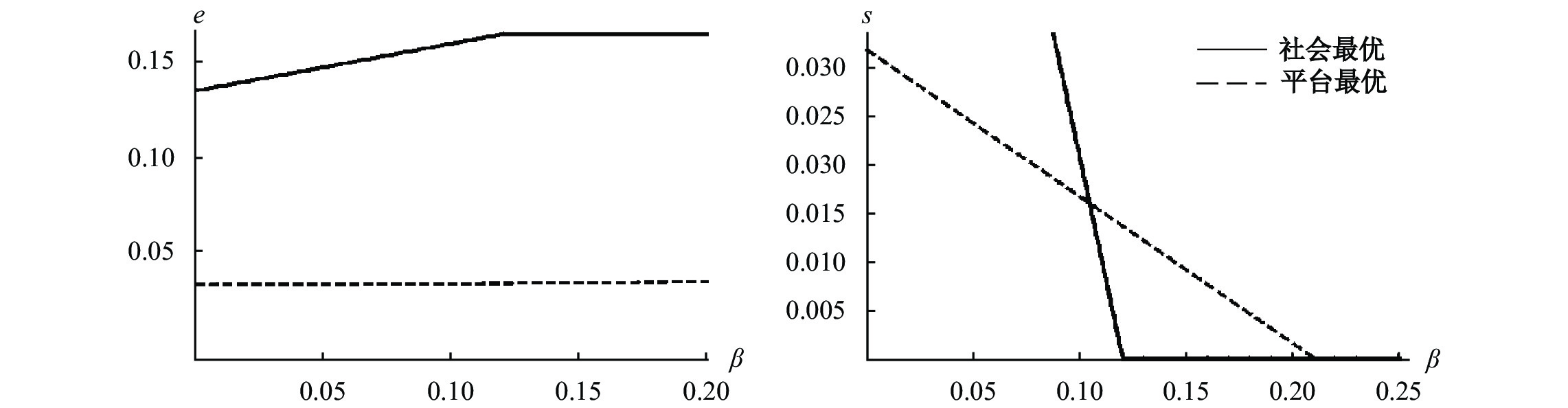图 1 $\mathit{\beta }$ 对 ${\mathit{e}}^{\mathbf{*}}{/\mathit{e}}^{\mathit{F}\mathit{B}}$ 和 ${\mathit{s}}^{\mathbf{*}}{/\mathit{s}}^{\mathit{F}\mathit{B}}$ 的影响

（四） ${k}_{s}$ 对均衡影响的对比分析。在 $\beta > 0$ 下， $s$ 在阻止 $b$ 类司机进入时必然会同时阻止部分 $g$ 类司机，平台和政府均更期望通过 $e$ 降低事故发生概率。下面将放松对 ${k}_{s}$ 的赋值，假定 $\dfrac{1}{3} < {k}_{s} < 1$ $\beta =0$ 。分析在 $s$ 阻止 $b$ 类司机完全有效且具有成本优势时， ${e}^{\mathrm{*}}{/e}^{FB}$ ${s}^{\mathrm{*}}{/s}^{FB}$ ${k}_{s}$ 的变化。此时平台最优下的均衡变为：

 ${s}^{\mathrm{*}}=\frac{38}{1200{k}_{s}-3}\text{，}{e}^{\mathrm{*}}=\frac{40{k}_{s}-2}{1200{k}_{s}-3}$ (21)

 ${s}^{FB}=\frac{26}{3(80{k}_{s}-9)}\text{，}{e}^{FB}=\frac{40{k}_{s}-11}{240{k}_{s}-27}$ (22)

${s}^{\mathrm{*}}$ ${s}^{FB}$ 均随 ${k}_{s}$ 的增加而减小； ${e}^{\mathrm{*}}$ ${e}^{FB}$ ${k}_{s}$ 的增加而增加，如图2所示：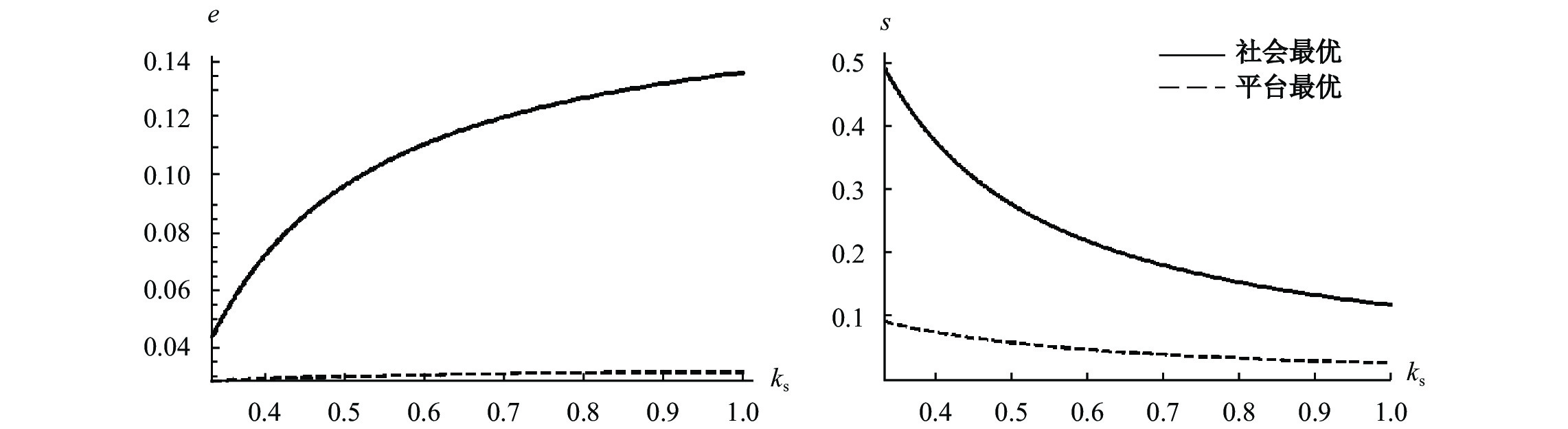图 2 ${\mathit{k}}_{\mathit{s}}$ 对 ${\mathit{e}}^{\mathbf{*}}{/\mathit{e}}^{\mathit{F}\mathit{B}}$ 和 ${\mathit{s}}^{\mathbf{*}}{/\mathit{s}}^{\mathit{F}\mathit{B}}$ 的影响

（五） ${k}_{e}$ 对均衡影响的对比分析。本小节剔除了司机对准入门槛的敏感性 $\beta$ 和进入成本系数 ${k}_{s}$ 的影响，令 $\beta =0，{k}_{s}=0$ 。进一步研究平台建设成本参数 ${k}_{e}$ ${e}^{\mathrm{*}}{/e}^{FB}$ ${s}^{\mathrm{*}}{/s}^{FB}$ 的影响。放松对 ${k}_{e}$ 的赋值，假定 $\dfrac{1}{3} < {k}_{e} < 1$ ${k}_{s}=0$ $\beta =0$ 。此时平台最优下的均衡为：

 ${s}^{\mathrm{*}}=\frac{2-40{k}_{e}}{3-1200{k}_{e}}\text{，}{e}^{\mathrm{*}}=\frac{38}{1200{k}_{e}-3}$ (23)

 ${s}^{FB}=\frac{10-36{k}_{e}}{15-228{k}_{e}}\text{，}{e}^{FB}=\frac{29}{3(76{k}_{e}-5)}$ (24)

${s}^{\mathrm{*}}$ ${s}^{FB}$ 均随 ${k}_{e}$ 的增加而增加； ${e}^{\mathrm{*}}$ ${e}^{FB}$ ${k}_{e}$ 的增加而减小，如图3所示：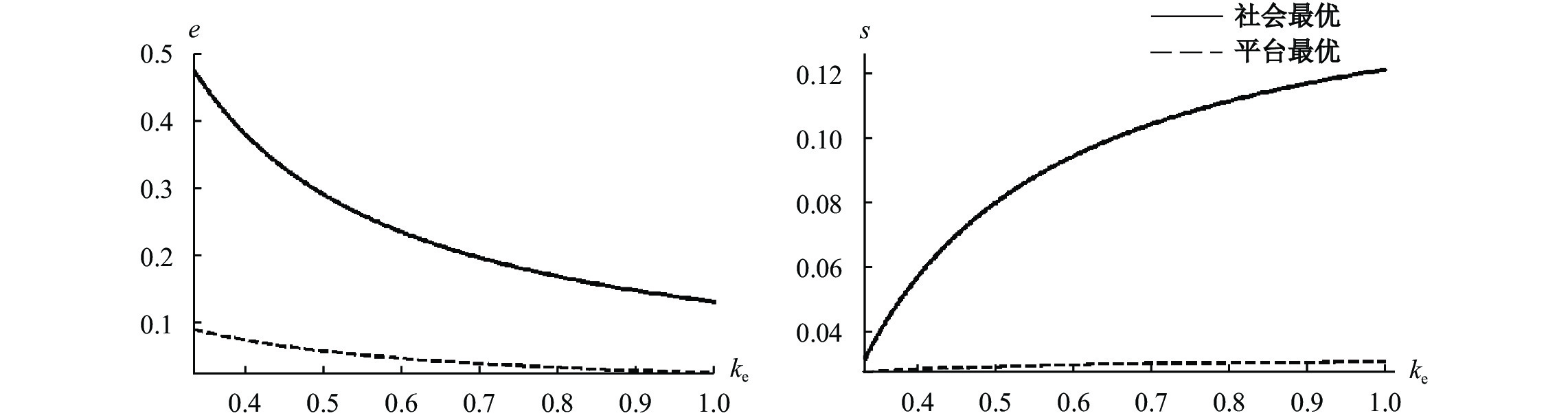图 3 ${\mathit{k}}_{\mathit{e}}$ 对 ${\mathit{e}}^{\mathbf{*}}{/\mathit{e}}^{\mathit{F}\mathit{B}}$ 和 ${\mathit{s}}^{\mathbf{*}}{/\mathit{s}}^{\mathit{F}\mathit{B}}$ 的影响

$SS=v\left({{n}}_{g}+{e}_{t}{\times {n}}_{b}\right)-H\left(1-{e}_{t}\right){{n}}_{b}-L\left(1-{e}_{t}\right){{n}}_{b}-[\left({n}_{g}\beta s+{n}_{b}\gamma s\right)+\left(\bar{c}\dfrac{{{n}_{g}}^{2}}{2\lambda }+$ $\bar{c}\dfrac{{{n}_{b}}^{2}}{2\left(1-\lambda \right)}\right)]- {k}_{s}{s}^{2}-{k}_{e}({{e}_{g}}^{2}+{{e}_{p}}^{2})$
，其中 ${e}_{t}={e}_{g}+{e}_{p}$ ${e}_{g}$ 为政府提供的安全建设投入， ${e}_{p}$ 为平台提供的安全建设投入。平台利润函数变为： ${\pi }=\alpha \left({{n}}_{g}+{{n}}_{b}\right)p-L\left(1-{e}_{t}\right){{n}}_{b}-{k}_{e}{{e}_{p}}^{2}-{k}_{s}{s}^{2}$ 。二者博弈顺序如下：第一阶段，政府根据社会福利最大化，自己提供令 $\dfrac{\partial SS}{\partial {e}_{g}}=0$ ${e}_{g}$ ；第二阶段，平台根据利润最大化，同时选择令 $\dfrac{\partial \pi }{\partial s}=0，\dfrac{\partial \pi }{\partial {e}_{p}}=0$ $s$ ${e}_{p}$

（一） $\beta$ 对监管政策的影响。三种政策下的均衡分别为：安全投入标准， ${s}_{1}={\left[\dfrac{599-3609\beta }{23457-27{\beta }^{2}}\right]}^{+} ，{e}_{1}=$ $Min[\dfrac{3658+1809\beta -18{\beta }^{2}+81{\beta }^{3}}{23457-27{\beta }^{2}}, \dfrac{1}{6}]$ ；司机准入标准， ${s}_{2}={\left[\dfrac{54-360\beta }{371-180{\beta }^{2}}\right]}^{+}，{e}_{2}=Min[\dfrac{29+54\beta -18{\beta }^{2}}{1113-540{\beta }^{2}},\dfrac{1}{30}]$ ；联合安全投入，

${s}_{3}={\left[\dfrac{5572-36000\beta }{233373-270{\beta }^{2}}\right]}^{+}，{e}_{3}={e}_{g}+{e}_{p}=Min[\dfrac{14714+6627\beta -60{\beta }^{2}+270{\beta }^{3}}{77791-90{\beta }^{2}},\dfrac{1}{5}]$
。平台最优、社会最优以及分别实施三种监管政策下的事故概率和社会福利如图4所示：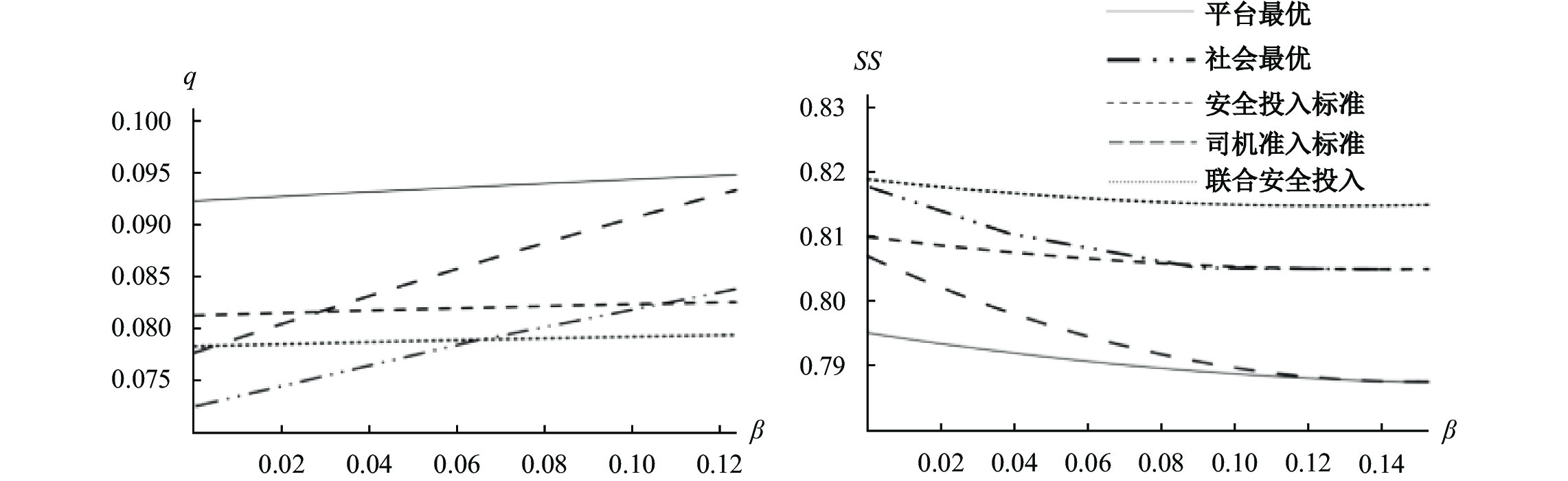图 4 β对监管政策的影响

（二） ${k}_{s}$ 对监管政策的影响。本节将在提供 $s$ 完全有效的情境下（ $\beta =0$ ），分析三种监管政策的有效性。三种政策下的均衡分别为：安全投入标准， ${s}_{1}=\dfrac{1-600{k}_{s}}{3+540{k}_{s}-24000{{k}_{s}}^{2}}，{e}_{1}=\dfrac{2+340 {k}_{s}-4000{{k}_{s}}^{2}}{3+540{k}_{s}-24000{{k}_{s}}^{2}}$ ；司机准入标准， ${s}_{2}=\dfrac{54}{400{k}_{s}-29}，{e}_{2}=\dfrac{11-40{k}_{s}}{87-1200{k}_{s}}$ ；联合安全投入， ${s}_{3}=\dfrac{28-5600{k}_{s}}{27+6600{k}_{s}-240000{{k}_{s}}^{2}}，{e}_{3}= {e}_{g}+$ ${e}_{p}= \dfrac{6+1280{k}_{s}-16000{{k}_{s}}^{2}}{9+2200{k}_{s}-80000{{k}_{s}}^{2}}$ 。平台最优、社会最优和三种政策下的事故概率和社会福利如图5所示：在提供 $s$ 完全有效的情境下，通过第三节的分析可以得知，在 ${k}_{s}$ 较小时， ${e}^{\mathrm{*}}$ ${e}^{FB}$ 之间的差距较小、 ${s}^{\mathrm{*}}$ ${s}^{FB}$ 之间的差距较大，此时政府应合理利用平台安全建设相对较强的激励，通过强制要求 $s$ 以弥补平台准入筛选不够严格的缺点。图5也反映出在 ${k}_{s} < 0.6$ 时，司机准入标准下的社会福利最大。随着 ${k}_{s}$ 的增加， ${e}^{\mathrm{*}}$ ${e}^{FB}$ 之间的差距逐渐扩大， ${s}^{\mathrm{*}}$ ${s}^{FB}$ 之间的差距逐渐缩小，平台设定严格司机准入门槛的激励逐渐增强，此时政府可以通过联合安全投入以弥补平台安全建设激励的不足。图5也反映出在 $0.6 < {k}_{s} < 0.85$ 时，联合安全投入下的社会福利最大。随着提供 $s$ 的效率优势进一步减弱，即 $0.85 < {k}_{s} < 1$ 时， ${e}^{\mathrm{*}}$ ${e}^{FB}$ 之间的差距进一步扩大， ${s}^{\mathrm{*}}$ ${s}^{FB}$ 之间的差距进一步缩小，此时政府再强制设定 $s$ 只会获得最低的社会福利，应通过联合安全投入或安全投入标准以保证有效的安全建设程度。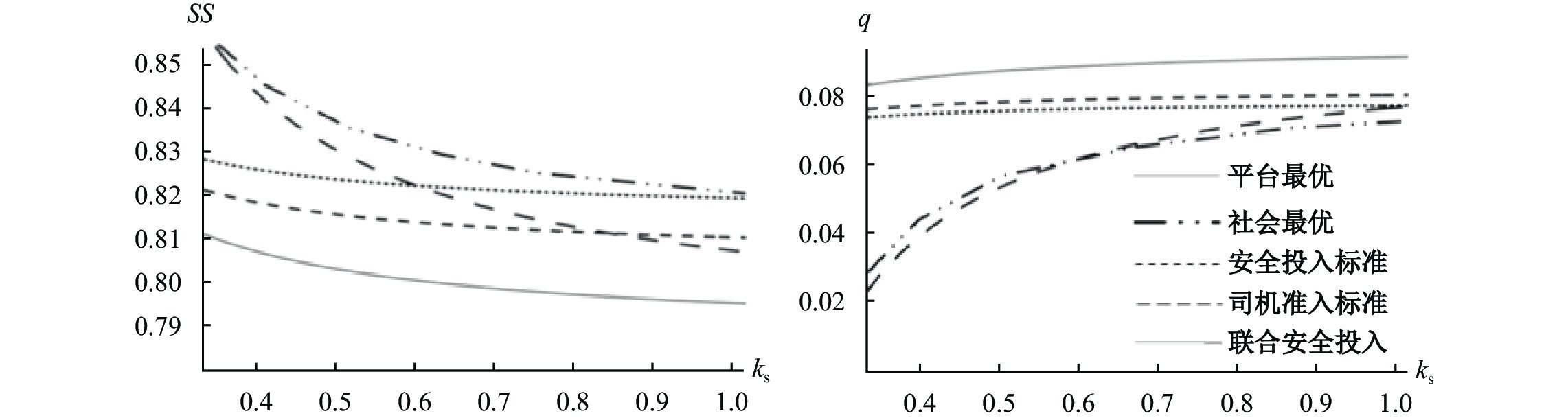图 5 ${\mathit{k}}_{\mathit{s}}$ 对监管政策的影响

① 资料来源：https://baijiahao.baidu.com/s?id=1650691678434007770&wfr=spider&for=pc，访问时间：2021.12.12。

② 2019年1月1日，《中华人民共和国电子商务法》正式实施；2019年8月，国务院专门出台了《关于促进平台经济规范健康发展的指导意见》。

③ 在 $\beta \ge \gamma$ 时，准入门槛不能被用于筛选排除更多的 $b$ 类司机，因而是无效的。本文在司机准入门槛有效的情况下进行探讨。

④ 通过改变 $H$ $L$ 的大小关系和减少 $\lambda$ 进行稳健性检验，结论较为稳健。具体检验可联系作者获取。

  方兴东, 严峰. 网络平台“超级权力”的形成与治理[J]. 人民论坛·学术前沿, 2019(14): 90–101.  刘奕, 夏杰长. 共享经济理论与政策研究动态[J]. 经济学动态, 2016(4): 116–125.  王俐, 周向红. 结构主义视阈下的互联网平台经济治理困境研究——以网约车为例[J]. 江苏社会科学, 2019(4): 76–85.  王小芳, 赵宇浩. 中国网约车规制政策述评[J]. 长安大学学报(社会科学版), 2016(3): 109–115. DOI:10.3969/j.issn.1671-6248.2016.03.019  汪旭晖, 张其林. 平台型网络市场“平台—政府”双元管理范式研究——基于阿里巴巴集团的案例分析[J]. 中国工业经济, 2015(3): 135–147.  汪旭晖, 张其林. 平台型电商企业的温室管理模式研究——基于阿里巴巴集团旗下平台型网络市场的案例[J]. 中国工业经济, 2016(11): 108–125.  王勇, 冯骅. 平台经济的双重监管: 私人监管与公共监管[J]. 经济学家, 2017(11): 73–80.  王勇, 刘航, 冯骅. 平台市场的公共监管、私人监管与协同监管: 一个对比研究[J]. 经济研究, 2020(3): 148–162.  吴德胜. 网上交易中的私人秩序——社区、声誉与第三方中介[J]. 经济学(季刊), 2007(3): 859–884.  肖红军, 李平. 平台型企业社会责任的生态化治理[J]. 管理世界, 2019(4): 120–144. DOI:10.3969/j.issn.1002-5502.2019.04.012  熊鸿儒. 我国数字经济发展中的平台垄断及其治理策略[J]. 改革, 2019(7): 52–61.  薛志远. 网约车数量管制问题研究[J]. 理论与改革, 2016(6): 108–113.  于洋, 马婷婷. 政企发包: 双重约束下的互联网治理模式——基于互联网信息内容治理的研究[J]. 公共管理学报, 2018(3): 117–128.  甄艺凯. 网约车管制新政研究[J]. 中国工业经济, 2017(8): 81–99.  Armstrong M. Competition in two-sided markets[J]. The RAND Journal of Economics, 2006, 37(3): 668–691. DOI:10.1111/j.1756-2171.2006.tb00037.x  Armstrong M, Wright J. Two-sided markets, competitive bottlenecks and exclusive contracts[J]. Economic Theory, 2007, 32(2): 353–380. DOI:10.1007/s00199-006-0114-6  Caillaud B, Jullien B. Chicken & egg: Competition among intermediation service providers[J]. The RAND Journal of Economics, 2003, 34(2): 309–328. DOI:10.2307/1593720  Farrell J, Katz M L. Innovation, rent Extraction, and integration in systems markets[J]. The Journal of Industrial Economics, 2000, 48(4): 413–432.  Gawer A, Cusumano M A. Platform leadership: How intel, microsoft, and cisco drive industry innovation[M]. Boston, MA: Harvard Business School Press, 2002.  Rochet J C, Tirole J. Platform competition in two-sided markets[J]. Journal of the European Economic Association, 2003, 1(4): 990–1029. DOI:10.1162/154247603322493212  Rochet J C, Tirole J. Two-sided markets: A progress report[J]. The RAND Journal of Economics, 2006, 37(3): 645–667. DOI:10.1111/j.1756-2171.2006.tb00036.x  Scott C. Private regulation of the public sector: A neglected facet of contemporary governance[J]. Journal of Law and Society, 2002, 29(1): 56–76. DOI:10.1111/1467-6478.00211